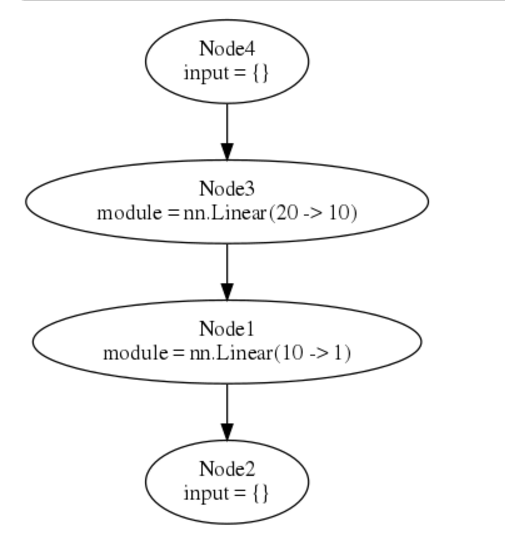# [learning torch] 5. nngraph (another way to construct nn)

[TOC]

The aim of this library is to provide users of nn package with tools to easily create complicated architectures.

`luarocks install nngraph`

optionally can install `graphvis` for graph visualization.

From previous posts, to build networks, there are 2 important classes:

• `Module` as a nn layer, can do forward and backward prop
• `Container` to combine several `Module`s

The nngraph library provides a way to build any complex network focusing on the network graph and avoiding the use of Containers.

## nngraph.Node

Any `Module` can be wrapped into a `nngraph.Node`.

For all `nn.Module`, nngraph overrides the `__call__` meta method (the function call operator `()`), by calling a module, a `Node` is returned:

```th> require 'nngraph';
[0.0000s]
th> module = nn.Identity()
[0.0001s]
th> module
nn.Identity
[0.0000s]
th> module()
nngraph.Node
[0.0000s]
```

The arguments of `__call__` are other (parent) nodes that come into this node, in this way we specify which module(s) will feed into the current node/module:

```th> h1 = nn.Linear(20, 10)()
[0.0001s]
th> h2 = nn.Linear(10, 1)(h1) -- h1 is input of h2
[0.0001s]
```

## Simple sequential example

We make a network by calling `nn.gModulet()`, this takes 2 arguments: a table of `inputs`, and a table of `outputs`:

```th> mlp = nn.gModule({h1}, {h2})
[0.0002s]
```

Then we can plot the model using graphviz:

```-- draw graph (the forward graph, '.fg'), use it with itorch notebook
graph.dot(model.fg, 'MLP')
-- or save graph to file MLP.svg and MLP.dot
graph.dot(model.fg, 'MLP', 'MLP')
```(The first and last nodes are dummy nodes and regroup all inputs and outputs of the graph.)

## DAG example

Here is an example to build a model with 2 inputs and 2 outputs:

```h1 = nn.Linear(20, 20)()
h2 = nn.Linear(10, 10)()
hh1 = nn.Linear(20, 1)(nn.Tanh()(h1))
hh2 = nn.Linear(10, 1)(nn.Tanh()(h2))
madd = nn.CAddTable()({hh1, hh2})
oA = nn.Sigmoid()(madd)
oB = nn.Tanh()(madd)
gmod = nn.gModule({h1, h2}, {oA, oB})

x1 = torch.rand(20)
x2 = torch.rand(10)

gmod:updateOutput({x1, x2})
gmod:updateGradInput({x1, x2}, {torch.rand(1), torch.rand(1)})
graph.dot(gmod.fg, 'Big MLP')
```

alternatively, use `-` to make your code looks like the data flow:

```h1 = - nn.Linear(20,20)
h2 = - nn.Linear(10,10)
hh1 = h1 - nn.Tanh() - nn.Linear(20,1)
hh2 = h2 - nn.Tanh() - nn.Linear(10,1)
madd = {hh1,hh2} - nn.CAddTable()
oA = madd - nn.Sigmoid()
oB = madd - nn.Tanh()
gmod = nn.gModule( {h1,h2}, {oA,oB} )
```

## Annotations of nodes

Use `node::annotate()` to annotated nodes, can add name/description, or change node color.

WIth annotation, can enable debugging too:

#### Part 5 of series «torch学习笔记»：

comments powered by Disqus